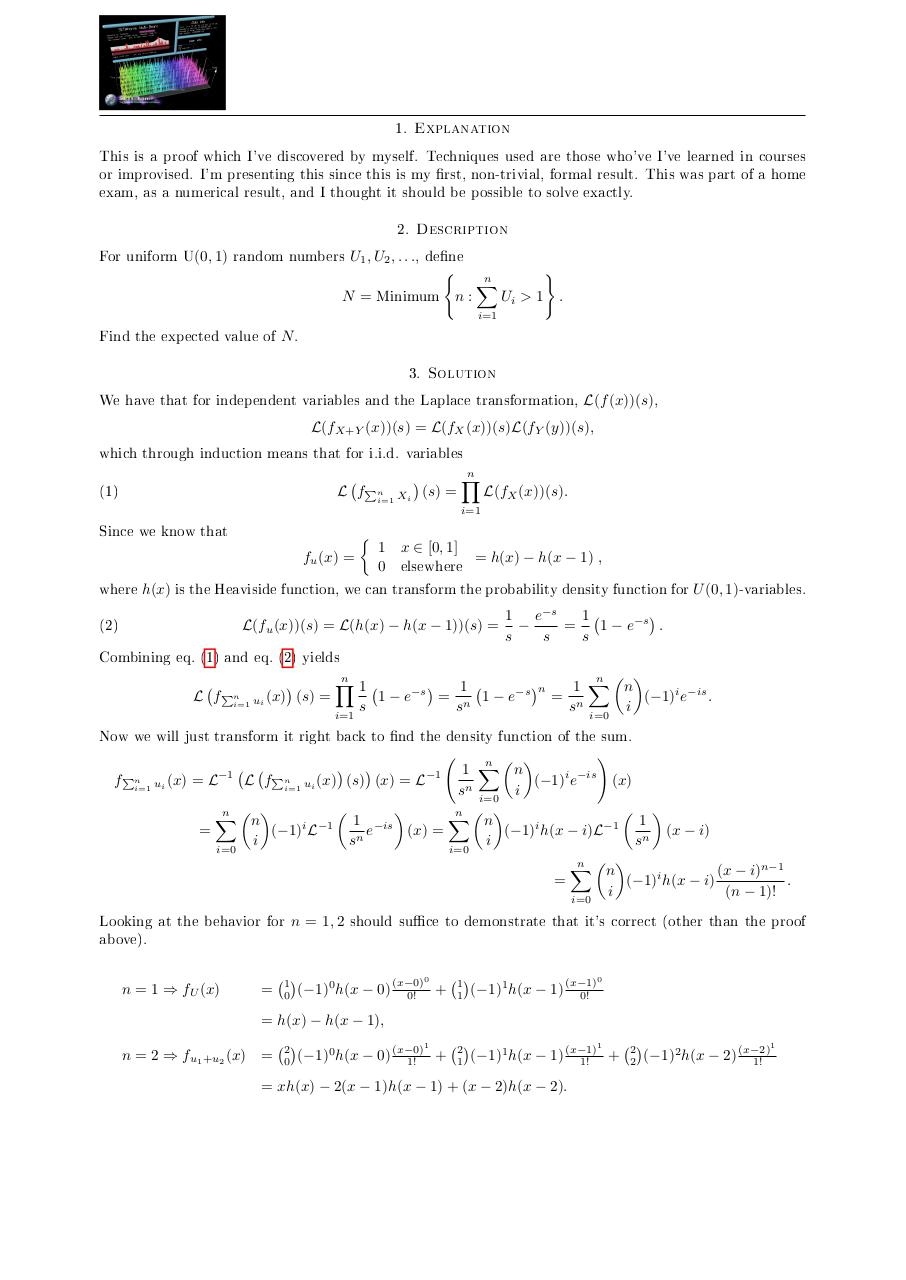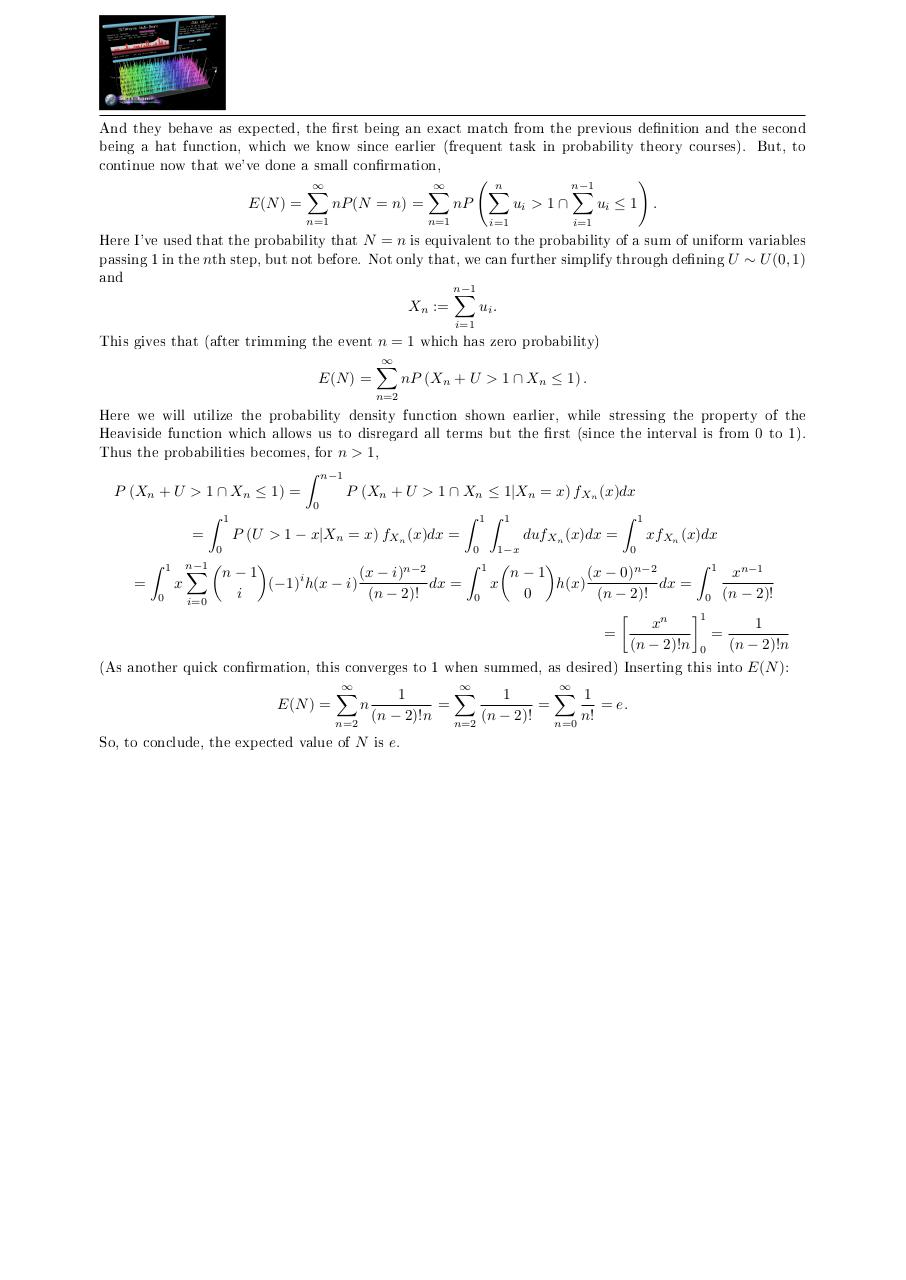# NiceProof p 2 3 .pdf

### File information

Original filename: NiceProof_p_2_3.pdf

This PDF 1.5 document has been generated by pdftk 2.02 - www.pdftk.com / itext-paulo-155 (itextpdf.sf.net-lowagie.com), and has been sent on pdf-archive.com on 30/10/2017 at 11:57, from IP address 130.239.x.x. The current document download page has been viewed 195 times.
File size: 323 KB (2 pages).
Privacy: public file

## Download original PDF file

NiceProof_p_2_3.pdf (PDF, 323 KB)

### Document preview

Explanation

1.

This is a proof which I've discovered by myself. Techniques used are those who've I've learned in courses
or improvised. I'm presenting this since this is my rst, non-trivial, formal result. This was part of a home
exam, as a numerical result, and I thought it should be possible to solve exactly.
2.

Description

For uniform U(0, 1) random numbers U1 , U2 , . . ., de ne
(
N = Minimum n :

n
X

)
Ui &gt; 1 .

i=1

Find the expected value of N .
3.

Solution

We have that for independent variables and the Laplace transformation, L(f (x))(s),
L(fX+Y (x))(s) = L(fX (x))(s)L(fY (y))(s),

which through induction means that for i.i.d. variables
n
Y

L fPni=1 Xi (s) =
L(fX (x))(s).

(1)

i=1

Since we know that

fu (x) =

1
0

x ∈ [0, 1]

= h(x) − h(x − 1) ,

elsewhere
where h(x) is the Heaviside function, we can transform the probability density function for U (0, 1)-variables.
(2)

L(fu (x))(s) = L(h(x) − h(x − 1))(s) =

1
1 e−s

=
1 − e−s .
s
s
s

Combining eq. (1) and eq. (2) yields
L

n
n
Y

1
1
1 X n
−s
−s n
(−1)i e−is .
1−e
= n 1−e
= n
(x) (s) =
i=1 ui
i
s
s
s
i=1
i=0

fPn

Now we will just transform it right back to nd the density function of the sum.
!
n
X
n
1
i
−is
fPni=1 ui (x) = L
(−1) e
(x)
sn i=0 i

n
n
X
X
n
1 −is
n
1
i −1
i
−1
=
(−1) L
e
(x) =
(−1) h(x − i)L
(x − i)
n
i
s
i
sn
i=0
i=0
n
X
n
(x − i)n−1
=
.
(−1)i h(x − i)
i
(n − 1)!
i=0
−1

L fPni=1 ui (x) (s) (x) = L−1

Looking at the behavior for n = 1, 2 should su ce to demonstrate that it's correct (other than the proof
above).
n = 1 ⇒ fU (x)

=

1
0

0

1
1

(−1)1 h(x − 1) (x−1)
0!

1

2
1

(−1)1 h(x − 1) (x−1)
+
1!

+
(−1)0 h(x − 0) (x−0)
0!

0

= h(x) − h(x − 1),
n = 2 ⇒ fu1 +u2 (x)

=

2
0

(−1)0 h(x − 0) (x−0)
+
1!

= xh(x) − 2(x − 1)h(x − 1) + (x − 2)h(x − 2).

1

2
2

(−1)2 h(x − 2) (x−2)
1!

1

And they behave as expected, the rst being an exact match from the previous de nition and the second
being a hat function, which we know since earlier (frequent task in probability theory courses). But, to
continue now that we've done a small con rmation,
E(N ) =

X

nP (N = n) =

n=1

X

n
X

nP

n=1

ui &gt; 1 ∩

i=1

n−1
X

!
ui ≤ 1 .

i=1

Here I've used that the probability that N = n is equivalent to the probability of a sum of uniform variables
passing 1 in the nth step, but not before. Not only that, we can further simplify through de ning U ∼ U (0, 1)
and
Xn :=

n−1
X

ui .

i=1

This gives that (after trimming the event n = 1 which has zero probability)
E(N ) =

X

nP (Xn + U &gt; 1 ∩ Xn ≤ 1) .

n=2

Here we will utilize the probability density function shown earlier, while stressing the property of the
Heaviside function which allows us to disregard all terms but the rst (since the interval is from 0 to 1).
Thus the probabilities becomes, for n &gt; 1,
Z

n−1

P (Xn + U &gt; 1 ∩ Xn ≤ 1) =

P (Xn + U &gt; 1 ∩ Xn ≤ 1|Xn = x) fXn (x)dx
0

Z

1

Z

0

Z
=

1

x
0

n−1
X
i=0

1

Z

1

P (U &gt; 1 − x|Xn = x) fXn (x)dx =

=

Z
dufXn (x)dx =

0

1−x

1

xfXn (x)dx
0

Z 1
Z 1
n−1
xn−1
(x − 0)n−2
n−1
(x − i)n−2
dx =
x
dx =
h(x)
(−1)i h(x − i)
(n − 2)!
0
(n − 2)!
i
0
0 (n − 2)!

1
xn
1
=
=
(n − 2)!n 0
(n − 2)!n

(As another quick con rmation, this converges to 1 when summed, as desired) Inserting this into E(N ):
E(N ) =

X
n=2

n

X
X
1
1
1
=
=
= e.
(n − 2)!n n=2 (n − 2)! n=0 n!

So, to conclude, the expected value of N is e.### Link to this page

#### Permanent link

Use the permanent link to the download page to share your document on Facebook, Twitter, LinkedIn, or directly with a contact by e-Mail, Messenger, Whatsapp, Line..

#### Short link

Use the short link to share your document on Twitter or by text message (SMS)

#### HTML Code

Copy the following HTML code to share your document on a Website or Blog

#### QR Code### Related keywords